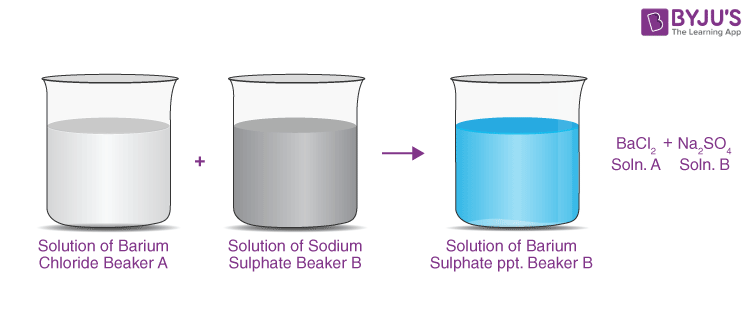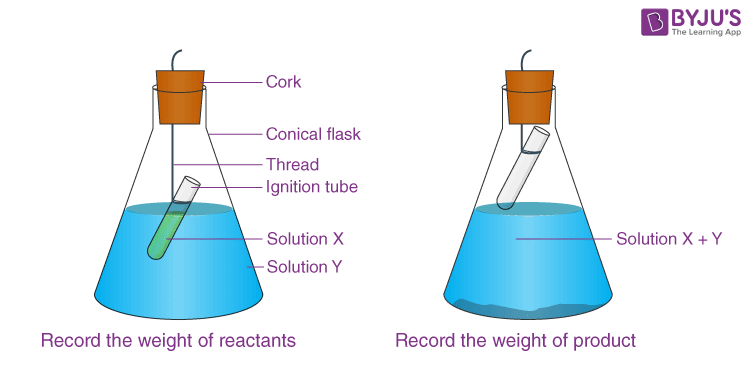Checkout JEE MAINS 2022 Question Paper Analysis : Checkout JEE MAINS 2022 Question Paper Analysis :

# Verification of the Law of Conservation of Mass in a Chemical Reaction

## Aim:

To verify the law of conservation of mass in a chemical reaction.

## Theory:

• In a chemical reaction, matter is neither created nor destroyed.
• The law of mass conservation states: Mass cannot be created or destroyed in a chemical reaction (except in nuclear reactions, where matter can be converted to energy).
• To make the product, the reactants —> atoms of one or more substances are purely rearranged.
• Total mass of reactants before reaction = Total mass of the product after reaction. Antoine Lavoisier proposed this law.
• Chemicals go through three types of transformations: physical, chemical, and nuclear.
• Physical change: Matter only undergoes phase transitions from solid to liquid and liquid to gas.
• Chemical change: Reactant atoms rearrange to produce new molecules.

$$\begin{array}{l}CaCO_{3} \xrightarrow[]{Heat} CaO + CO_{2}\end{array}$$

• Nuclear change: An atom’s nucleus can be modified by increasing or decreasing the number of protons in it, or by splitting the nucleus. Uranium, for example, can fission to produce Barium and Krypton.
• When reactants react chemically, heat may be produced or released, gas may be released, bubbles may develop, colour may change, or precipitate may form.

## Materials Required:

Two watch glasses, beakers, weighing balance and glass rod.

## Chemicals Required:

Distilled water and one of the following sets of chemicals.

Set I → Copper sulphate and Sodium carbonate

Set II → Barium chloride and Sodium sulphate

Set III → Lead nitrate and Sodium chloride

(X)(Y)

## Procedure:

1. Take two watch glasses and weigh them on the balancing scale.
2. In a watch glass of known mass, weigh 3.6g of BaCl2.2H2O.
3. Pour 50 mL of distilled water into a 100 mL beaker. Beaker ‘A’ should be labelled.4. In beaker ‘A,’ dissolve the weighed BaCl2.2H2O.
5. Now weigh 8.05g of Na2SO4.H2O in a known-mass watch glass and dissolve it in the other beaker holding 50 ml of distilled water. Label the beaker as ‘B.’
6. Take a second beaker. Weigh it and mark it with the letter ‘C.’
7. In beaker C, combine the contents of beakers A and B. With a glass rod, stir it.
8. The synthesis of (BaSO4) Barium Sulphate results in the formation of a white precipitate in beaker ‘C.’
9. Weigh beaker ‘C’ again with the product you obtained and keep track of your findings.
10. Subtract the mass of beaker ‘C’ before and after adding the solutions from ‘A’ and ‘B’ beakers to get the mass of the finished product.

## Observations:

• Mass of Reactants = 44 g
• Mass of Products = 44 g
• Mass of BaCl2.2H2O = 3.6 g
• Mass of BaCl2, solution = 53.6 g
• Mass of Na2SO4.10H2O = 8.05 g
• Mass of Na2SO4 solution = 58.05 g
• Total mass of reactants is 53.6 g + 58.05 g = 111.65 g (BaCl2, soln.) (Na2SO4 solution.)
• Mass of beaker ‘C’ is C1 = 500 g
• Mass of reaction mixture on adding in beaker ‘C’ is C2 = 611.65 g
• Mass of the product formed = C2 – C1 = 111.65 g

Note: Mass of 50 ml of distilled water = 50.0 g

(density of water = 1 g/mL)

## Result:

Thus, Initial mass of reactant = Final mass of the product (reactant mixture).

$$\begin{array}{l}BaCl_{2}(aq) + Na_{2}SO_{4}(aq) \to BaSO_{4}(s) + 2NaCl(aq)\end{array}$$

## Procedure:

1. Make a 5% solution of any of the X and Y compounds listed.
2. In a conical flask, place a small amount of Y solution and a small amount of X solution.
3. As illustrated in the diagram, carefully suspend the ignite tube in the flask. The test tube solution must not flow into the flask.
4. Cover the flask with a cork.
5. Carefully weigh the flask and its contents.
6. Now gently tilt and swirl the flask to combine the solutions X and Y.
8. Make a note of your findings.
9. Note the mass of the conical flask before reaction.## Observations:

• Initial weight of conical flask + ignition tube with X and Y solutions =……g
• Weight of conical flask + ignition tube when solution X and Y are mixed and reaction has taken place =……. g.
• The mass of the reactants equals the mass of the products.

## Result:

• Mass of reactants equals mass of products
• In a chemical reaction, there is no mass loss.

## Precautions:

1. When using the weighing machine, use caution.
2. Only use distilled water to make the solution.
3. Do not taste any chemical.
4. When solution X and Y have been combined, place a cork in the bottle.
5. To calculate the resultant mass of the product, subtract the mass of the conical flask + cork.
6. When the chemicals (reactants) are mixed, cork should be used to prevent the gas, or vapours, from escaping. The law is verified only in closed systems.
7. While recording the initial mass, be sure the chemical does not run out of the ignition tube.

## Chemical Reactions:

• Set I :
$$\begin{array}{l}CuSO_{4} + Na_{2}CO_{3} \to Na_{2}SO_{4} + CuCO_{3}\end{array}$$
• Set II :
$$\begin{array}{l}BaCl_{2} + Na_{2}SO_{4} \to BaSO_{4} + 2NaCl\end{array}$$
• Set III :
$$\begin{array}{l}Pb(NO_{3})_{2} + 2NaCl \to 2NaNO_{3} + PbCl_{2}\end{array}$$